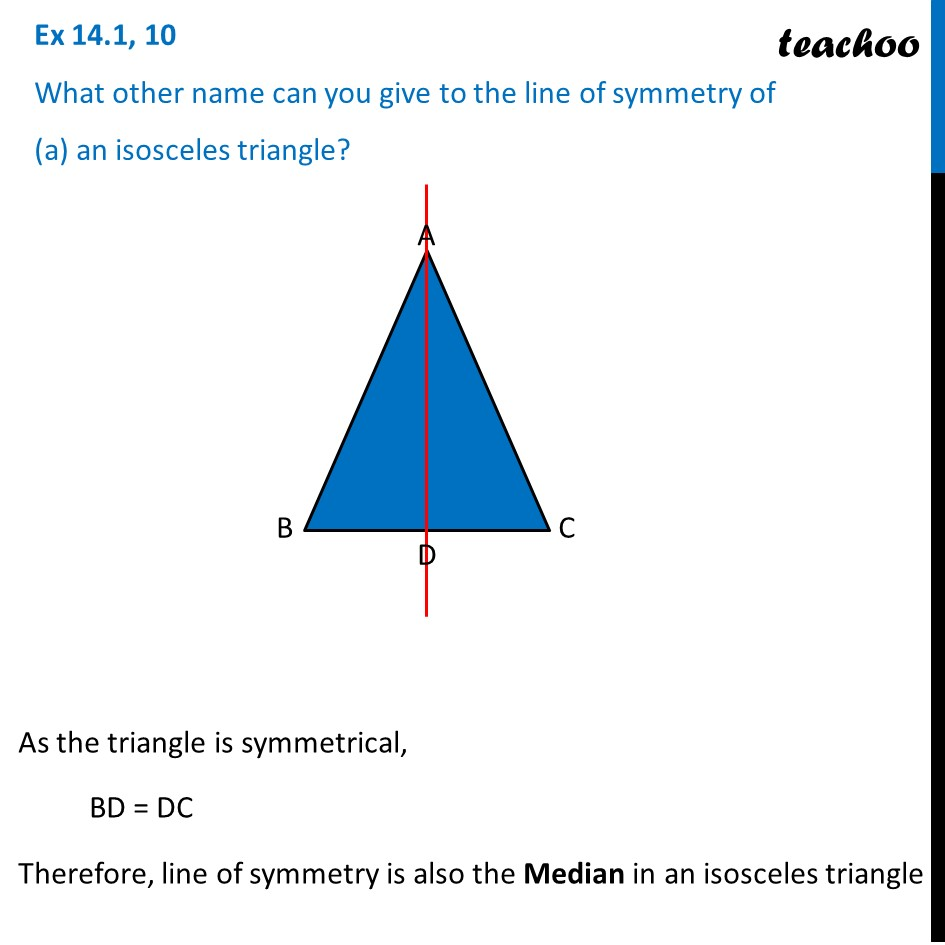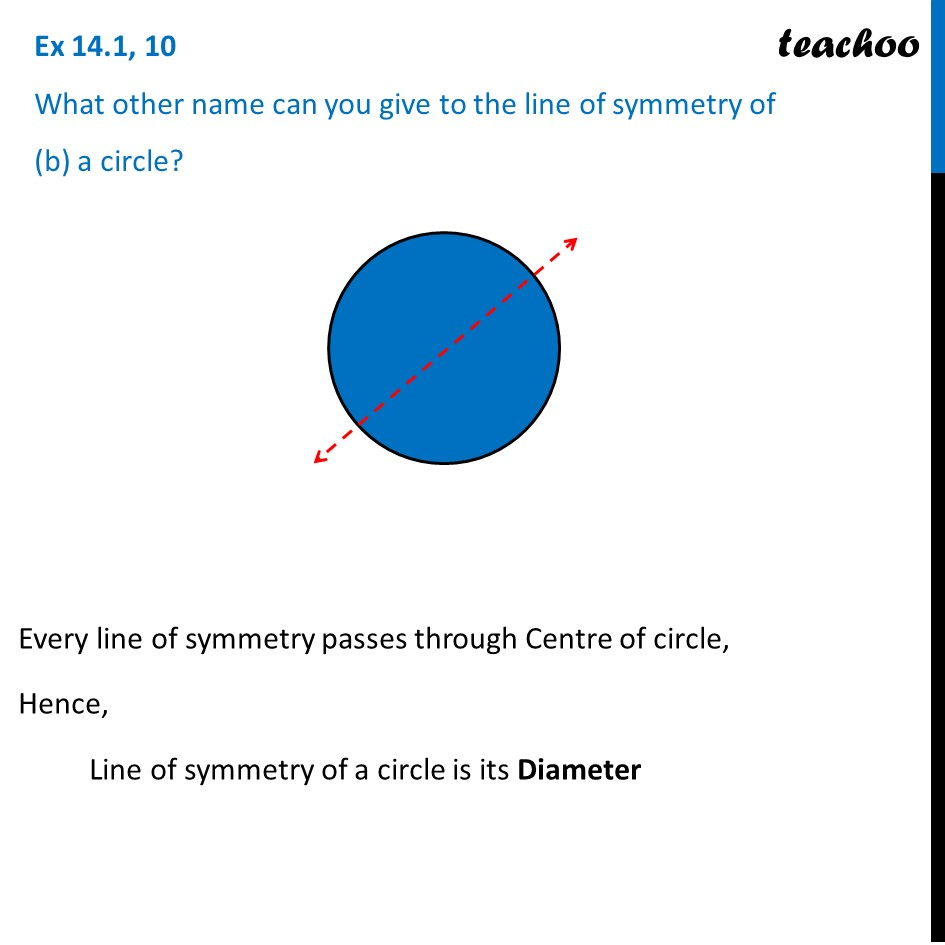Multiple lines of symmetry

Chapter 14 Class 7 Symmetry
Concept wiseIntroducing your new favourite teacher - Teachoo Black, at only ₹83 per month

### Transcript

Ex 14.1, 10 What other name can you give to the line of symmetry of (a) an isosceles triangle? As the triangle is symmetrical, BD = DC Therefore, line of symmetry is also the Median in an isosceles triangle Ex 14.1, 10 What other name can you give to the line of symmetry of (b) a circle? Every line of symmetry passes through Centre of circle, Hence, Line of symmetry of a circle is its Diameter